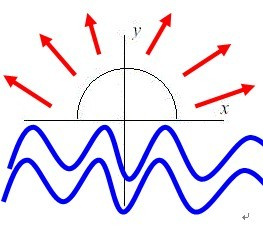# POJ 1005 - I Think I Need a Houseboat

## 问题描述

Fred Mapper 正在考虑在路易斯安那州购买一些土地来建他自己的房子。在研究土地的过程中，他发现，路易斯安那州的土地每年都会被密西西比河侵蚀掉 50 平方里。因为 Fred 希望在这个房子里度过余生，所以他需要知道他的那些土地是否会被侵蚀掉。## 解题思路

Fred Mapper 的家 `(x, y)` 到侵蚀中心 `(0, 0)` 的距离就是侵蚀半径 R

## AC 源码

``````/*
Author:     Exp
Date:       2017-11-29
Code:       POJ 1005
Problem:    I Think I Need a Houseboat
URL:        http://poj.org/problem?id=1005
*/

/*
根据题意建立数学模型如下：
位于平面坐标第一二象限的一个半圆从原点(0,0)开始向(x,y)扩散，每次扩散50平方
求第几次扩散会覆盖到(x,y)， 其中y>0

勾股定理半径 R^2 = (x-0)^2 + (y-0)^2
半圆面积公式 Area = pi * R^2 / 2
当 Area 是 50 整数倍时, 扩散次数 cnt = Area / 50
当 Area 不是 50 整数倍时, 扩散次数 cnt = Area / 50 + 1    （亦即向上取整）
*/

#include <math.h>
#include <iostream>
using namespace std;

const static double PI = 3.141592654;    // 常量π
const static double HALF_PI = PI / 2;    // 半圆面积公式常量
const static double EACH_AREA = 50;    // 每次扩散面积

/*
* 求解扩散次数
* @param x 扩散坐标x
* @param y 扩散坐标y
* return 扩散次数
*/
int diffuse(double x, double y);

int main(void) {
int testCase = 0;    // 测试用例数
cin >> testCase;

for(int i = 1; i <= testCase; i++) {
double x, y;
cin >> x >> y;
int cnt = diffuse(x, y);
cout << "Property " << i << ": This property will begin eroding in year " << cnt << '.' << endl;
}
cout << "END OF OUTPUT." << endl;

//system("pause");
return 0;
}

int diffuse(double x, double y) {
double R2 = x * x + y * y;
double Area = HALF_PI * R2;
return (int) ceil(Area / EACH_AREA);    // 向上取整
}``````

目录